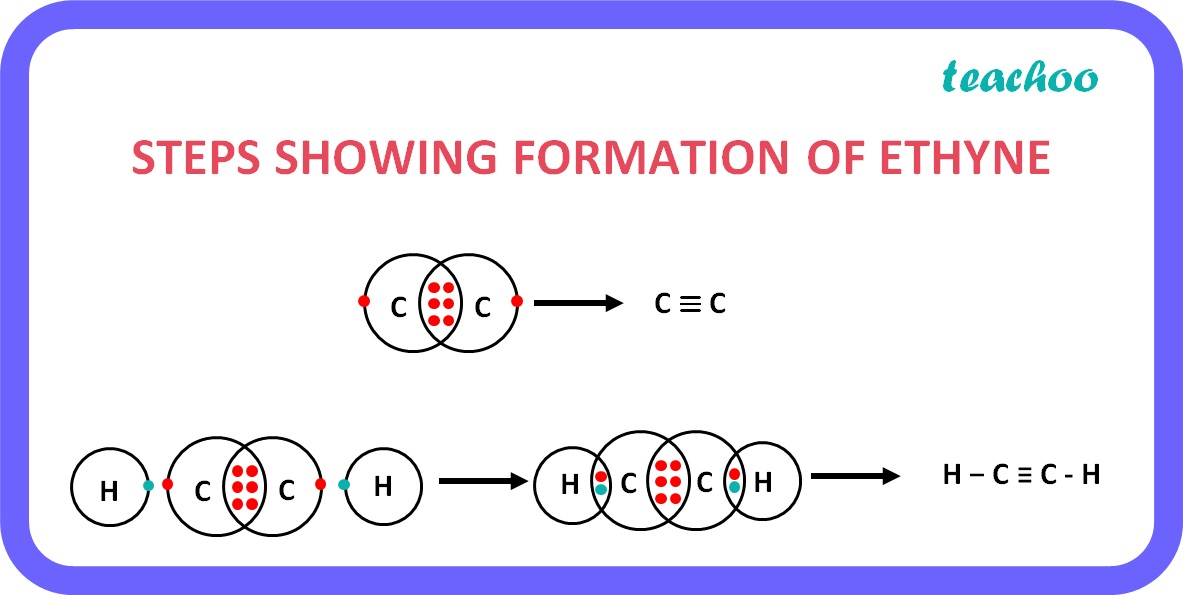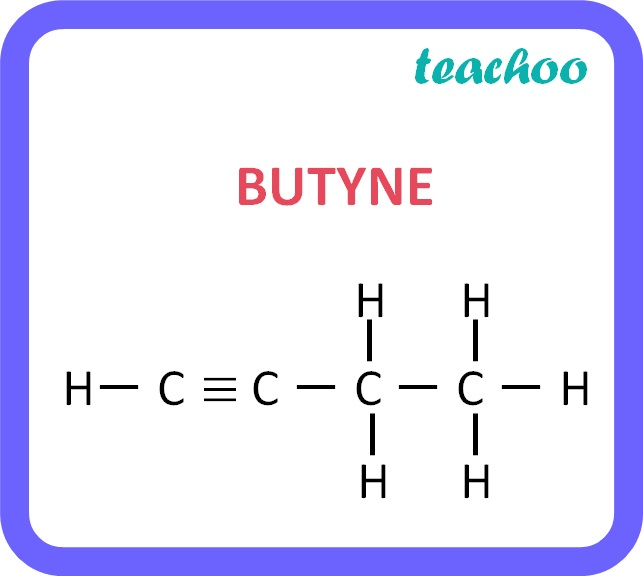Concepts

Class 10
Chapter 4 Class 10 - Carbon and its Compounds

## Alkynes -

### What are Alkynes:

These are the hydrocarbons in which carbon atoms are connected by triple bonds .

### Example: Ethyne

• Formula of Ethyne is C 2 H 2
• There is a triple bond between 2 carbon atoms.
• Since a carbon atom has 4 valence electrons and 3 of them are shared by another carbon atom, the remaining 1 electron is shared by a hydrogen atom each.
• Hence there are a total of 1+1=2 Carbon Atoms and 1+1=2 hydrogen atoms .### General formula for Alkynes

It Is C n H 2n-2

• Where n is the number of carbon atoms.

 Number of Carbon Atoms (n) n=2 n=3 n=4 Name of Alkyne Ethyne Propyne Butyne Structure

### Example : Butyne

• In butyne, there are 4 carbon atoms.
• So, the formula is:

C n H 2n-2

• Putting n=4,

C 4 H 2*4-2

C 4 H 8-2

C 4 H 6Name of Alkyne Formula Derivation C n H 2n-2 Number of carbon atoms (n) Ethyne C 2 H 2 n=2 Propyne C 3 H 4 n=3 Butyne C 4 H 6 n=4 Pentyne C 5 H 8 n=5 Hexyne C 6 H 10 n=6 Heptyne C 7 H 12 n=7 Octyne C 8 H 14 n=8 Nonyne C 9 H 16 n=9 Decyne C 10 H 18 n=10

Learn in your speed, with individual attention - Teachoo Maths 1-on-1 Class